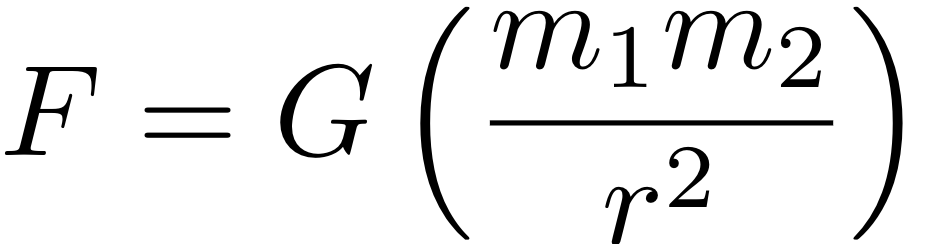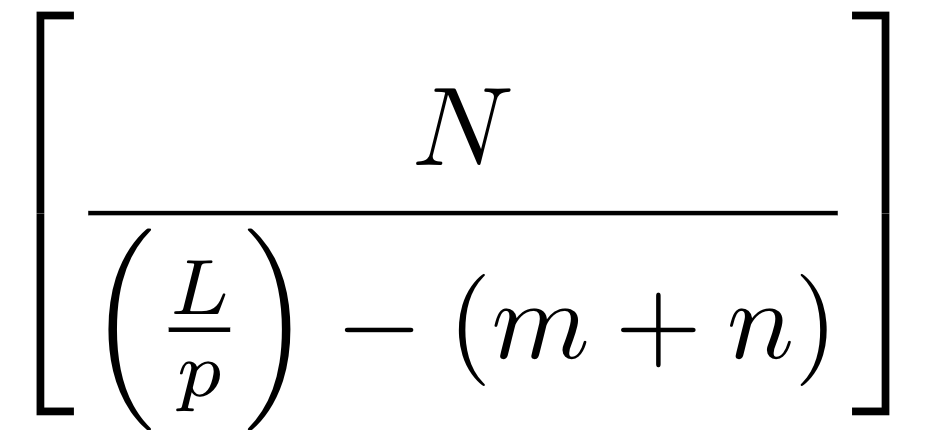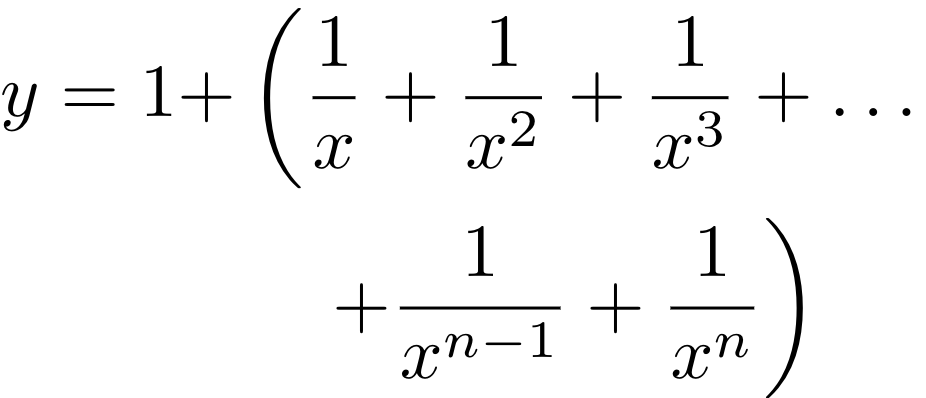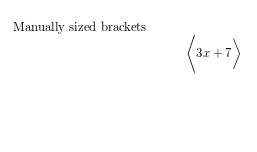Showing first {{hits.length}} results of {{hits_total}} for {{searchQueryText}}{{hits.length}} results for {{searchQueryText}}

No Search Results

Parentheses and brackets are very common in mathematical formulas. You can easily control the size and style of brackets in LaTeX; this article explains how. Contents 1 Introduction 2 Controlling types and sizes 3 Reference guide 4 Further reading Introduction Here's how to type some common math braces and parentheses in LaTeX: Type LaTeX markup Renders as Parentheses; round brackets (x+y) $$(x+y)$$ Brackets; square brackets [x+y] $$[x+y]$$ Braces; curly brackets \{ x+y \} $$\{ x+y \}$$ Angle brackets \langle x+y \rangle $$\langle x+y\rangle$$ Pipes; vertical bars |x+y| $$\displaystyle| x+y |$$ Double pipes \|x+y\| $$\| x+y \|$$ The size of brackets and parentheses can be manually set, or they can be resized dynamically in your document, as shown in the next example: $F = G \left( \frac{m_1 m_2}{r^2} \right)$Notice that to insert the parentheses or brackets, the \left and \right commands are used. Even if you are using only one bracket, both commands are mandatory. \left and \right can dynamically adjust the size, as shown by the next example: $\left[ \frac{ N } { \left( \frac{L}{p} \right) - (m+n) } \right]$When writing multi-line equations with the align, align* or aligned environments, the \left and \right commands must be balanced on each line and on the same side of &. Therefore the following code snippet will fail with errors: $y = 1 + & \left( \frac{1}{x} + \frac{1}{x^2} + \frac{1}{x^3} + \ldots \\ & \quad + \frac{1}{x^{n-1}} + \frac{1}{x^n} \right)$ The solution is to use "invisible" brackets to balance things out, i.e. adding a \right. at the end of the first line, and a \left. at the start of the second line after &: $y = 1 + & \left( \frac{1}{x} + \frac{1}{x^2} + \frac{1}{x^3} + \ldots \right. \\ & \quad \left. + \frac{1}{x^{n-1}} + \frac{1}{x^n} \right)$Controlling types and sizes The size of the brackets can be controlled explicitly Manually sized brackets $\Bigg \langle 3x+7 \bigg \rangle$The commands \Bigg and \bigg stablish the size of the delimiters < and > respectively. For a complete list of parentheses and sizes see the reference guide. Reference guide LaTeX markup Renders as \big( \Big( \bigg( \Bigg( ${\big (}{\Big (}{\bigg (}{\Bigg (}$\big] \Big] \bigg] \Bigg] ${\big ]}{\Big ]}{\bigg ]}{\Bigg ]}$\big\{ \Big\{ \bigg\{ \Bigg\{ ${\big \{}{\Big \{}{\bigg \{}{\Bigg \{}$\big \langle \Big \langle \bigg \langle \Bigg \langle ${\big \langle }{\Big \langle }{\bigg \langle }{\Bigg \langle }$\big \rangle \Big \rangle \bigg \rangle \Bigg \rangle ${\big \rangle }{\Big \rangle }{\bigg \rangle }{\Bigg \rangle }$\big| \Big| \bigg| \Bigg| $$\displaystyle\big| \; \Big| \; \bigg| \; \Bigg|$$ \big\| \Big\| \bigg\| \Bigg\| $$\displaystyle\big\| \; \Big\| \; \bigg\| \; \Bigg\|$$ \big \lceil \Big \lceil \bigg \lceil \Bigg \lceil $$\displaystyle\big \lceil \Big \lceil \bigg \lceil \Bigg \lceil$$ \big \rceil \Big \rceil \bigg \rceil \Bigg \rceil $$\displaystyle\big \rceil \Big \rceil \bigg \rceil \Bigg \rceil$$ \big \lfloor \Big \lfloor \bigg \lfloor \Bigg \lfloor $$\displaystyle\big \lfloor \Big \lfloor \bigg \lfloor \Bigg \lfloor$$ \big \rfloor \Big \rfloor \bigg \rfloor \Bigg \rfloor $$\displaystyle\big \rfloor \Big \rfloor \bigg \rfloor \Bigg \rfloor$$ Further reading Mathematical expressions Subscripts and superscripts Aligning equations with amsmath Display style in math mode Operators The not so short introduction to LaTeX2ε